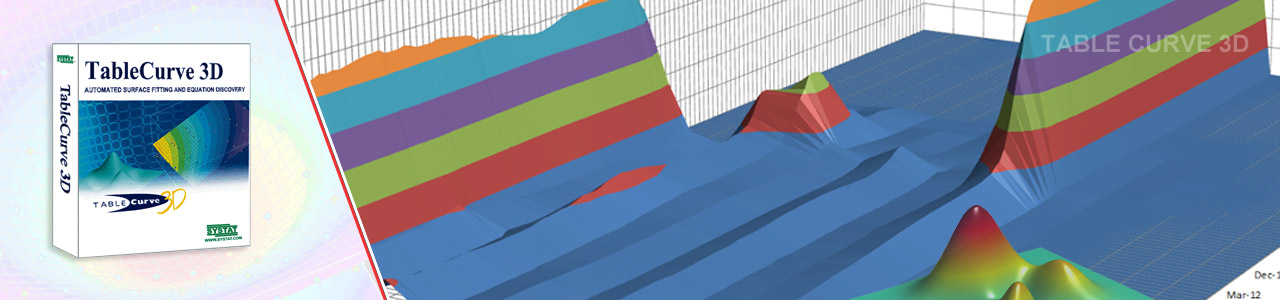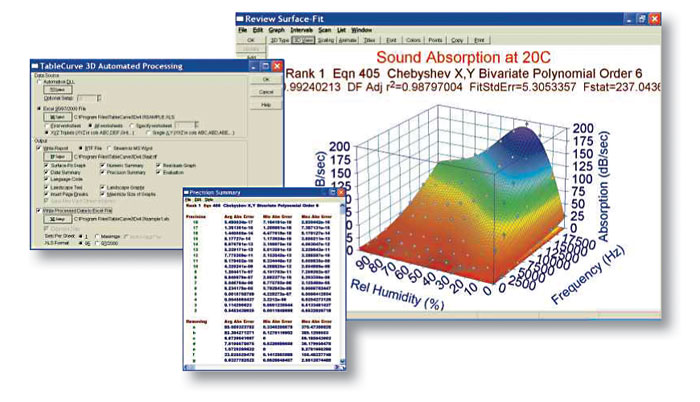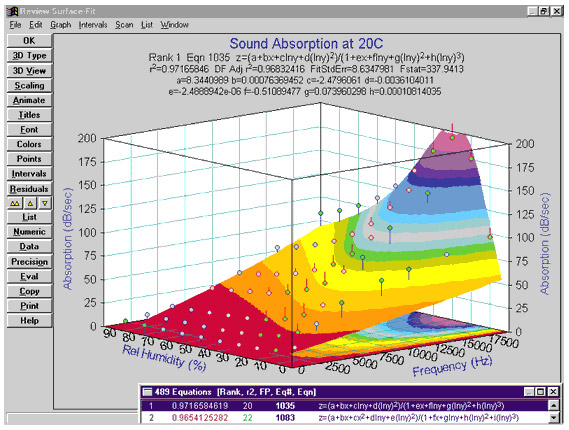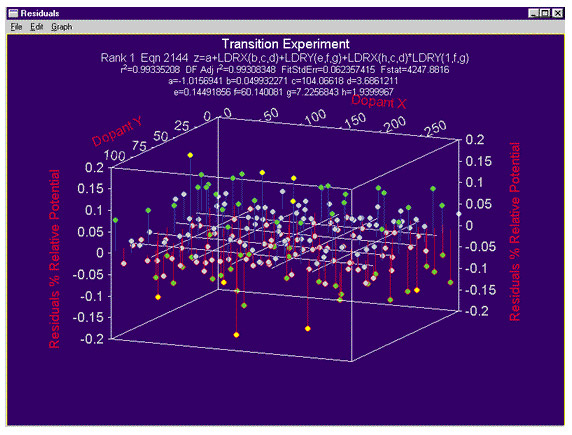TableCurve 3DTableCurve 3D – Model Complex Data Sets Fast and EasyEliminate Tedious Data Analysis Chores with TableCurve 3D

TableCurve 3D is the first and only program that combines a powerful surface fitter with the ability to find the ideal equation to describe three dimensional empirical data.

TableCurve 3D uses a selective subset procedure to fit 36,000 of its 453,697,387 built-in equations from all disciplines to find the one that provides the ideal fit – instantly!

What once could take days of tedious work now takes minutes, with a much more powerful result.

TableCurve 3D® gives scientists and engineers the power to find the ideal model for even the most complex data, including equations that might never have been considered. TableCurve 3D’s built-in equation set includes a wide array of linear and nonlinear models for any application:

• Linear equations
• Polynominal and rational functions
• Logrithmic and exponential functions
• Nonlinear peak functions
• Nonlinear transition functions
• Nonlinear exponential and power equations
• User-defined functions (up to 15)

TableCurve 3D’s state-of-the-art surface fitting includes capabilities not found in other software packages:

• In addition to standard least squares minimization, TableCurve 3D’s non-linear engine is capable of three different robust estimations: least absolute deviation, Lorentzian minimization and Pearson VII Limit minimization.
• Option to change the maximum number of terms permitted when fitting linear equations (minimum 3; maximum 11)
• On systems that support multi-threading, TableCurve 3D’s Background Thread Processing option allows fitting to occur without any form of user input
• Option to set the default term significance anywhere from 1 to 15.Using its selective subset procedure, TableCurve 3D will fit 36,000 of the over 450 million built-in equations or just the ones you need – instantly. With TableCurve 3D, a single mouse click is all it takes to start the automated curve fitting process – there is no set up required! You can even enter your own specialty models to be fit and ranked along with the built-in equations. TableCurve saves you precious time because it takes the endless trial and error out of curve fitting.Up to 15 user-defined equations can be entered and ranked along with the built-in equations. These specialized models can contain most mathematical constructs, including special functions, series convergence and conditional statements, differentiations, integrations and parameter constraints. TableCurve 3D even offers the option of graphically adjusting equation parameters to assure convergence for the fit of user-defined models. Unlike most surface fitting programs, TableCurve 3D’s user-defined functions are compiled so they can be fitted at nearly the speed of the built-in equations. For maximum flexibility, TableCurve 3D gives you the option to save your functions as individual files, in libraries or both.

Once your XYZ data have been fit, TableCurve 3D automatically sorts and plots the fitted equations by the statistical criteria you select (r2, DOF adjusted r2, Fit Standard Error or the F Statistic). Graphically review the fitted results as you scroll through the equation list. A 3D residuals graph as well as parameter output are generated for each fitted equation. Add confidence or prediction intervals to the graph to detect outliers in your data. You can also automatically display a 2D contour plot on the top and bottom of the surface fit graph to get another view of your data. Data, statistical and numeric summaries are also available from within the Review Surface Fit window so you can further analyze fit results.Viewing a surface fit from all angles is imperative in determining whether or not a given fit is accurate. Using a simple interface, TableCurve 3D lets you view a graph from any angle. It will even animate the graph automatically in a specified XY and/or Z angle sequence. Just sit back and observe every nuance within the fit. TableCurve 3D gives you all of the tools you need to discover the model that best meets your requirements for the ideal fit.

Output TableCurve 3D’s publication-quality graphs in black and white or color, portrait or landscape. You can also produce files containing data and equations in Lotus, Excel, ASCII, Quattro Pro and SigmaPlot formats. TableCurve 3D can speed up your programming by generating actual function code and test routines for all fitted equations in FORTRAN, C, Basic and Pascal.TableCurve 3D takes full advantage of the Windows graphical user interface to simplify every aspect of operation – from data import to output of results. Import data from many popular file formats including SigmaPlot, Excel, Lotus, SPSS and ASCII. Once your data are in the TableCurve editor, start the automatic fitting process with a single mouse click. Choose to fit all equations, select a group of equations or create a custom equation set. All equations are readily available from the Toolbar or TableCurve’s Process Menu. You can even set up TableCurve 3D to begin fitting the moment data are imported or modified with Background Thread Processing Fitting. Users consistently comment that – out of the box, without reading the instructions – TableCurve is highly intuitive, easy-to-use and remarkably simple to learn.